Benedikt Bitterli
• Home
• Portfolio
• Resume
• Resources

## Star Stacker: Astrophotography with C++11

If you've ever taken a photo of the night sky with a cell phone or a consumer camera, you might have been disappointed with the results. The problem is that stars are actually surprisingly dim, and to properly capture them on film, the camera sensor needs to be exposed to the sky for a long time. However, most consumer cameras use only a short exposure time, and you might end up with a picture that looks like the raw input image in the video above: A dim image with only a handful of bright stars visible - certainly not worth sharing on your social medias.

Since I'm a programmer and not a photographer, the obvious question to ask was this: Maybe we can't take better pictures - but if we had a lot of such low quality input images, could we somehow extract a single, higher quality picture of the night sky from them? This is what this project is all about.

If we throw plenty of math at the problem, the answer is yes! - with enough input images, we can reconstruct hundreds of stars from what used to be a black night sky. However, getting there turns out to be quite difficult. In the following, I will briefly outline my approach to the problem, what worked and what didn't work. There will also be plenty of pretty pictures along the way.

## Disclaimer

Before I dive into the details of this project, I should add a quick disclaimer. I am neither a photographer nor a computer vision person, and my approach to solve this problem may be clumsy at times. But I wanted to play with computer vision for a while now, and it was a refreshing side project to work on. To that end, most of the code was written from scratch, since I wanted to avoid big dependencies like OpenCV - it's not much of a learning experience otherwise. If you have any suggestions or corrections to make, feel free to contact me.

It's also worth mentioning that these things are not new. There are already software packages that can enhance your star photos for you (pixinsight, DeepSkyStacker, AstroArt, just to name a few). The problem I found with these is that they are either quite expensive or performed substandard when it comes to aligning images. It's possible that these just need more manual preprocessing and dialing in the right settings before they produce better results, but this is unsatisfying: My goal was to have a piece of software that won't need any user adjustment or complicated setup to work. After all, what is the fun in having a program do the work for you when you have to spend just as much time setting it up!

## Overview

The basic problem we're trying to solve is that we have a photo of something that's very dim taken with a short exposure, and as a result we can't see anything. Our first instinct is to simply turn up the image brightness, and this is almost the correct thing to do. If we do this to our input image, we get a result like this: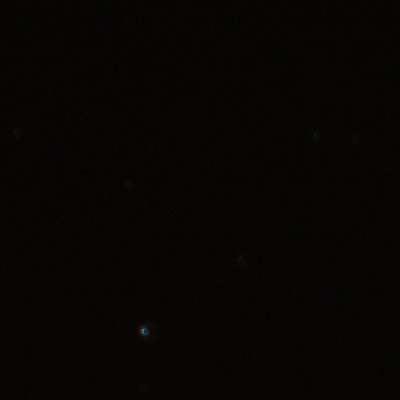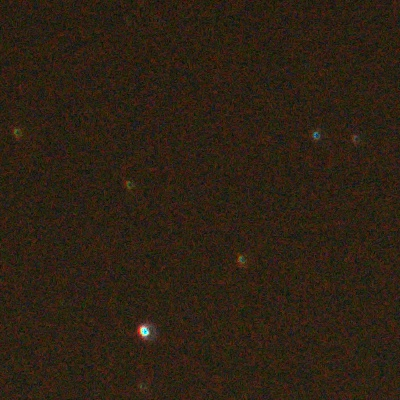If we take an input star image (left) and just increase its brightness, we don't get great results (right).

There are three problems here: Our color channels only have 8 bits of precision, so we can't just make the image brighter and expect more detail to appear. Additionally, multiplying the image brightness also multiplies the noise level and leaves us with nasty red and green image speckles in the result. Finally, making the image brighter also reveals the problem of light pollution: City lights that get reflected by the atmosphere and occlude the stars we want to see, leading to an orange film in front of the night sky: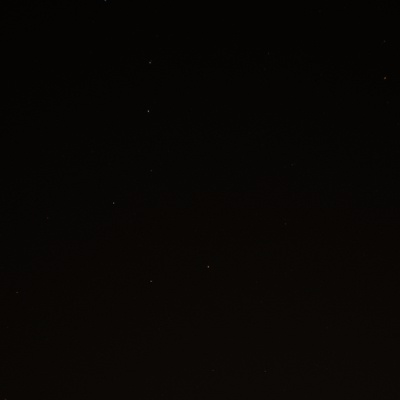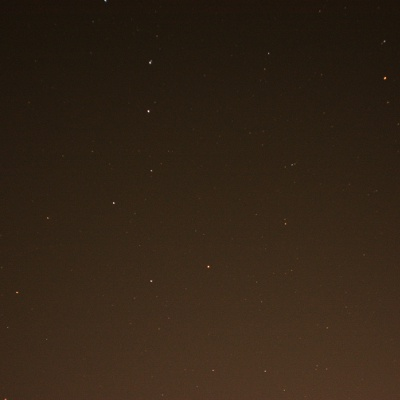Increasing the image brightness reveals the light pollution that occludes the night sky.

## Removing light pollution

Even if we can remove noise and increase precision, light pollution puts a hard limit on how much we can boost the image brightness; eventually, we'll just end up with a white image. Of course, the ideal solution is to avoid the problem in the first place, i.e. drive far away from civilization and take photos unpolluted by man-made lights. But that's a non-software solution. Let's instead try to get the most out of the photos we have!

What helps us is that light pollution looks completely different to the stars we want to extract: Stars are tiny bright dots, and light pollution is a smoothly changing tint over the whole image. Using these properties, we can build a simple process to remove light pollution: First, we will come up with a mathematical model that can express "smoothly changing tint" as a simple formula with few parameters. Then, we build a crude guess of which pixels contain stars and which are part of the background. We can then fit the parameters of the formula so that it best matches the background of the image. If all goes well, the fitted formula then closely predicts the value of light polution over the whole image. Then we can simply subtract the fitted model from the input, and what is left should hopefully be just stars.

Coming up with a formula that can describe light pollution over the whole image is quite difficult, and we're not going to do that. Instead, we're going to use a classic modelling trick: We will use a very simple formula, but only use it to model a small patch of pixels (say, a 50x50 pixel square). Then we simply use an ensemble of many such simple formulas to cover the whole image. Simple is better than complex, and I'm going to assume that we can model light pollution within a small square of pixels as a linear gradient.

Our first step in removing light pollution is to build a binary mask that roughly classifies pixels into "probably stars" and "probably background". This mask does not have to be perfect - as long as we can exclude most of the stars, we will be fine.

I'm going to use the fact that light pollution is smooth and low frequency, whereas stars are small dots significantly brighter than the background. This means that if we take the average brightness over a small patch of pixels, then background pixels will be "close" to the average, whereas stars will be a lot brighter.

We need to be careful how we measure the "closeness" of two brightness values. Our input is polluted by camera noise and has an unknown dynamic range, which means that hardcoding an absolute threshold is going to be fragile and will only work for some inputs. Instead, we're going to model our background pixels to be polluted by Gaussian noise. To use this model, we estimate the mean and sample variance of all pixels within a small image patch surrounding the pixel we want to classify. We then compare the target pixel to the mean; if its difference to the mean is smaller than two standard deviations, the fluctuation can likely be explained as noise and we classify the pixel as "probably background". A larger deviation suggests this pixel cannot be explained by our model for background pixels and we classify it as "probably stars".

If we do this for our input image, we get a mask as shown below: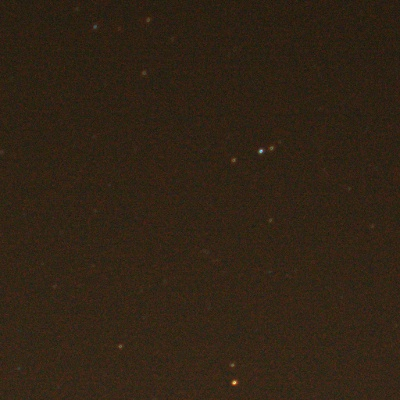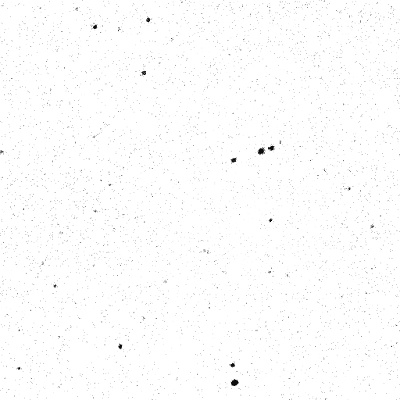An input image (left) with a rough binary mask (right) classifying pixels into stars (black) and background (white).

We can see some spurious background pixels that get classified as stars, but overall the result looks quite good. It will definitely suffice for our purposes.

### Estimating the light pollution

Now that we have roughly classified unwanted star pixels, we can fit our model for light pollution to the remaining background pixels. As mentioned before, we model light pollution within small patches of pixels as a linear gradient. Such a gradient has three parameters: Its brightness, its x-slope and its y-slope. Our goal now is to estimate these parameters so that the linear gradient best matches the input image.

This problem is best known as Weighted Least Squares and is well-studied. We can write the optimal fit in terms of a set of linear equations $$(\mathbf{X}^T \mathbf{W} \mathbf{X}) \beta = \mathbf{X}^T \mathbf{W} y,$$ where $y$ is the vector of input pixels, $\beta$ are the parameters of our model, $\mathbf{X}$ is the feature matrix (pixel positions + a column of constant 1s) and $\mathbf{W}$ is a diagonal matrix containing our image mask. This will not make much sense unless you are familiar with linear regression (I won't go into the details), but just assume that this allows us to compute the optimal parameters. Arbitrary sized matrix math is inconvenient to implement, and I've opted to use the excellent Eigen matrix library to solve this system of equations.

Once we have estimated the parameters $\beta$, we can go in the reverse direction: Evaluating $X \beta$ retrieves the value of the model for all pixels in the patch. If we do this over the whole image, this is what we get: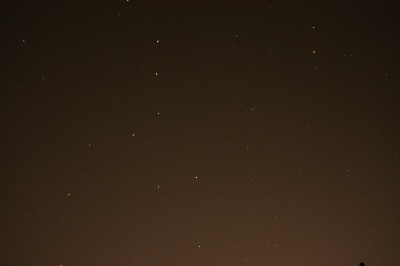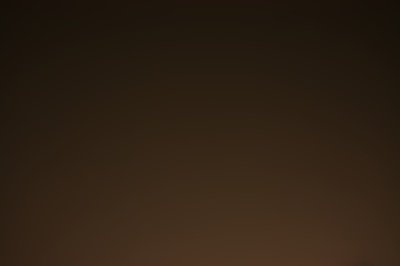An input image (left) and its model of light pollution (right) estimated from the input using weighted linear least squares.

Not too bad! Here's what happens when we subtract our model from our input image: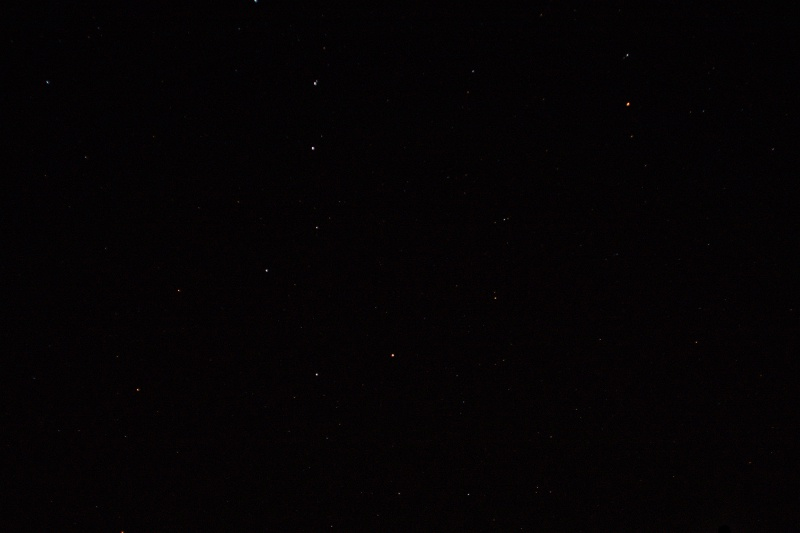Subtracting our fitted model of light polution from the input reveals a clean, black night sky with stars left intact.

This is exactly what we were after. The light pollution is completely gone, but our stars were left intact. And all that with just software - no need to leave the comfort of our bedroom.

## Stacking Frames

With a single image, this is about as good as it gets. With light pollution removed, we can boost the image brightness by a bit and extract a few more stars. However, we still struggle with a lack of dynamic range and speckles of camera noise, which only get worse the brighter we make the image.

The solution here is to use not just one, but multiple images taken a short time apart. With some assumptions, the noise in different images is uncorrelated and just fluctuates around zero - i.e. sometimes pixels come out darker and sometimes brighter than they should be, but on average we get the correct value.

By the law of large numbers, if we had a lot of pictures of the same scene and averaged them, we will get a noise-free and very precise result of what the scene actually looks like.

Let's try this for a sequence of images taken of the night sky: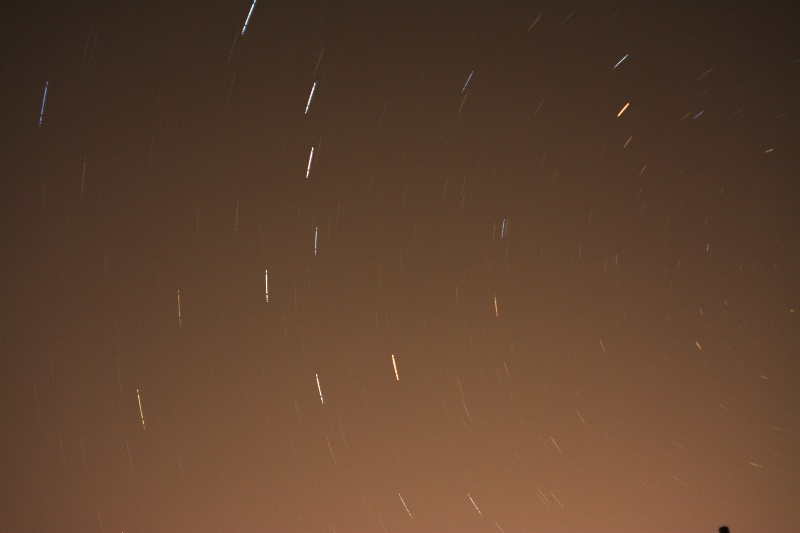Naively averaging an input sequence smears stars into star trails.

Woah! This looks nothing like our input image. What we're seeing here is an effect called star trails. Even though the camera was static, the earth moved while we were taking pictures. This causes stars to wander between pictures and leave trails if we naively average the images.

While this certainly looks cool, it's not the effect we want. Because the stars move between exposures, we won't get more accurate pixel data for each star when we average images - all we get is a smeared version of the star.

The solution to this problem needs a bit more math. The basic idea is that we need to undo the rotation of the earth and align all the pictures with each other before we average them. This will make it possible to extract a much more precise image of each star. We don't know the exact rotation, so we need to estimate it from the images. To do this, we will need several steps; here's a rough outline:

• Star extraction: We first need to precisely locate all the stars in the image. These are our reference points that we want to track between images.
• Coarse alignment: We then need to match stars between images and compute a rough initial guess for how the images are rotated with respect to each other. This algorithm needs to be fast and robust, but it doesn't need to be too accurate.
• Fine alignment: With each frame roughly aligned to the next frame and correspondences between stars established, we run a more sophisticated optimization algorithm to precisely align frames.
• Merging: After all frames are finely aligned, we subtract light pollution and transform each image through a camera model. This allows us to average the aligned images and obtain a merged output.
The reason why we need both a rough and fine alignment step is that the fine alignment algorithm I'm using will fail spectacularly unless the frames are already fairly close to each other. To solve this problem, we first run a completely different rough alignment algorithm that gets us most of the way there, while we use fine alignment to get precisely matching images.

## Finding stars

The first step in our alignment pipeline is to precisely locate stars in an input image. In computer vision this falls under the umbrella term of "feature extraction", which extracts "trackable" features from an image. There's a wide range of established algorithms to do this, such as SIFT, SURF, GLOH and HOG, just to name a few.

However, I am not going to use any of these. Stars have very few distinctive features (they're just white blobs), and these general-purpose algorithms would be overkill. Additionally, unlike these general-purpose methods, we can leverage specific knowledge about our stars to compute their location very precisely. And finally, it's a lot more educational to build something of our own than to implement someone else's ideas.

### Blob transform

We know that stars are more or less white blobs in a dark background. Therefore, the first step in extracting stars is to try and locate "blob-like" objects in the image. This is not easy when operating on the RGB data directly, and we will instead pre-process our image using an algorithm called Difference of Gaussians

The basic idea is that we take an input image, blur it slightly and subtract the blurred image from the input. In uniform regions, the blur won't change much and the output will be zero. However, close to edges and corners, the blurring will change the image significantly, and the magnitude of the difference will be large. For blob-like objects, the difference of gaussians algorithm will react particularly strongly, which makes it very useful for our purposes.

Another cool thing is that we can repeat the blurring and subtracting to obtain a whole chain of filtered outputs - this allows us to detect blobs of different sizes. I've illustrated this below for an input image of a Cornell box:An illustration of the Difference of Gaussians on a Cornell box image.

From left to right, the amount of blur increases, and the size of features detected by the algorithm increases accordingly. As an optimization, you would usually combine a blur step with a downsampling step (e.g. halve the image size after blurring), which results in what is called a Gaussian pyramid.

### Rough star extraction

After running the difference of gaussians, we end up with a stack of grayscale images. If a pixel in one of these images has a large value, it means that at that location in the input image, there was a blob-like object. For a fixed pixel location, we can also look at all the images in the stack so check which one has the brightest value at that point. This tells us the size of the blob: Images obtained from larger blurs capture larger blobs, and vice-versa. The exact formula for the radius can be obtained from the blur parameters.

To make these images useful, we now need to convert the pixel data into a concrete list of blob positions and radii. We're interested in the most "blob-like" features in the input, which corresponds to finding local maxima in the image stack. I'm going to use a very simple algorithm for this: First, I will pick the most blob-like feature (i.e. the brightest pixel) in the entire image stack, and add it to my list of potential stars. Then I will remove all other blobs that overlap with the one I picked. This amounts to going through all images in the stack and blacking out a circle with the radius of the blob we picked. We then simply repeat this process until we've obtained a sufficient number of stars.

I've illustrated a run of this algorithm on an example input image. The estimated blob locations and radii are shown on the right: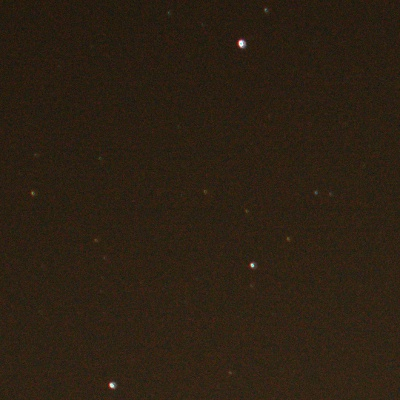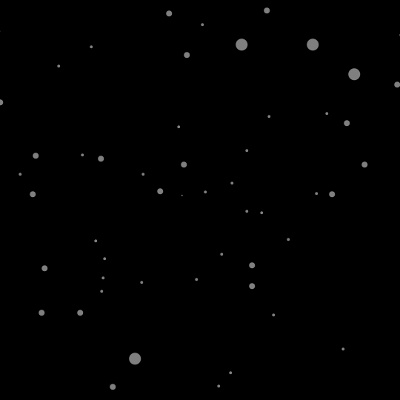An input image (left) and its roughly estimated star positions and radii (right).

The results look quite decent. It manages to extract all directly visible stars, as well as a lot of extremely dim stars that are tough to detect by eye. There are also some false positives, but we can filter these out later.

### Precise star extraction

The algorithm described above will give us a rough initial guess of where the stars in the image are. The extracted star positions are very inaccurate however, which is unfortunate - we want to use the stars as fixpoints to align images, so we need the positions to be as accurate as possible.

To do this, we will refine the star positions with a second algorithm. We will use the knowledge that stars are very much blob-like. This means they can be well approximated with a 2D Gaussian: A controllable "blob function". To illustrate, here's a heatmap of a 2D Gaussian with some hand-picked parameters: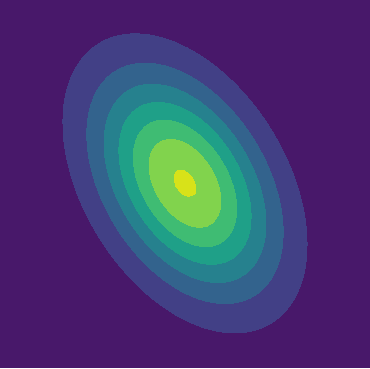The nice thing about Gaussians is that, given a 2D point set, we can directly compute the parameters of a Gaussian that best fits the input data. This allows us to create the following refinement algorithm: We use the rough blob positions as an initial guess, and extract a small image patch (say, 64x64 pixels) around each blob position. If the initial guess is correct and there is a star close to that blob, then we should be able to reproduce it well with a Gaussian. Therefore, as a next step we fit a 2D Gaussian that best fits the image patch we extracted. The mean value of the Gaussian is the star position.

If we apply this to a sample input, we obtain an image like this:An input image (left) and a naive Gaussian estimation of star location and shapes.

Awful! These Gaussians do not reproduce the stars well at all. The problem here is that the input image is polluted by a constant level of background noise. A constant value added to a blob generally cannot be modelled with a 2D Gaussian, and as a result the best fit ends up being an extremely large Gaussian that tries to account for both the noise and the star.

However, we can just apply the same trick here that we used earlier for subtracting the light pollution. We first measure the mean and variance within the image patch; anything that falls within a few standard deviations of the background is most likely noise and should be ignored when fitting the Gaussian. If we apply this in a weighted Gaussian fitting scheme, we get this result: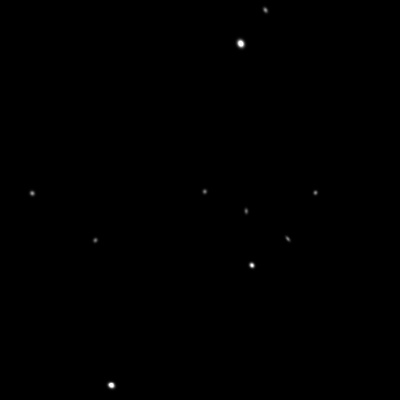An input image (left) and corresponding star Gaussians (right) estimated using a model accounting for background noise.

This is really quite good!

The great thing is that fitting the Gaussian uses the information of a whole neighborhood of pixels, and the resulting estimated star position has sub-pixel accuracy. This algorithm has a few other desirable properties too, such as being robust with respect to rotation and noise. This ensures we will be able to accurately track stars across a whole sequence of images

#### Pruning

Our initial coarse detection step is bound to have a few false positives, and we should filter these out in a final pruning step. We can use a lot of information from the Gaussian fit to determine whether there really was a star or not. If the Gaussian has a very large radius, or is very dim, then we likely just captured a piece of the background and should remove this star.

### Camera Model

With a list of precise star locations computed for each image, we are starting to have enough data to start aligning images in our sequence. But before we figure out how we do this, we first need to discuss what exactly it is that we are doing.

In order to make image alignment easy, we are first going to make a few assumptions. Our first assumption is that stars are infinitely far away, which means that there is no parallax: If we move the camera, nothing will change in the image; only when things rotate do we start to see change. This way, we don't have to worry about solving for camera translation. Additionally, we'll assume that the stars stay fixed in place while the camera (or, rather, the earth) rotates. Finally, we'll assume our camera is well-behaved: It has a centered image plane and negligible distortion.

In a camera model, we're interested in relating 3D points, i.e. $v = (X, Y, Z)$, to points on the camera image plane, i.e. $p = (x, y, 1)$. We can do this using the transformation $$p \propto \mathbf{V} \mathbf{R} v,$$ where $$\mathbf{V} = \begin{bmatrix} f & 0 & 0 \\ 0 & f & 0 \\ 0 & 0 & 1 \end{bmatrix}$$ is the intrinsic camera matrix and $\mathbf{R}$ is the extrinsic camera matrix. The intrinsic matrix describes properties of the camera apparatus, such as distortion and offset. In our case, we only include the camera's focal length (described by the $f$ parameter). The extrinsic matrix describes the camera pose, which in our case reduces to a rotation matrix.

Note that I've used the proportional sign rather than an equality in the projection equation above. This is because we will do a perspective division after the linear transformation. We first compute $(\overline{x}, \overline{y}, \overline{z}) = \mathbf{V} \mathbf{R} v$, and then obtain $p = (\overline{x}/\overline{z}, \overline{y}/\overline{z}, \overline{z}/\overline{z}) = (x, y, 1)$.

I will make the reasonable assumption that all the images in our sequence were taken by the same camera, which means we will have to deal with only a single intrinsic matrix. However, each image will have a different camera pose. We will index the images in our sequence and assign a rotation matrix to each; frame $l$ gets orientation $\mathbf{R}_l$ and so forth. In order to align images, we need to solve for an orientation $\mathbf{R}_l$ for all frames, and a single global $f$ parameter.

To do this, we'll frequently make use of image correspondences. An image correspondence is a single 3D point that appears in two different frames - for example, a star that has been captured by two images in the input sequence. Let's call our 3D point $v$, and its projections $p_l$ and $p_k$ in frames $l$ and $k$ of the input sequence. We can relate these points with \begin{align} p_l &= \mathbf{V} \mathbf{R}_l v \\ p_k &= \mathbf{V} \mathbf{R}_k v . \end{align} Of course, if we just have the images, then we don't know $v$ in general. However, if we know that $p_l$ and $p_k$ are projections of the same point, then we can rewrite the equations above into \begin{align} p_l &= \mathbf{V} \mathbf{R}_l \mathbf{R}_k^{-1} \mathbf{V}^{-1} p_k \\ &= \mathbf{V} \mathbf{R}_{lk}^{-1} \mathbf{V}^{-1} p_k, \end{align} where $\mathbf{R}_{kl}$ is the relative pose between frames $k$ and $l$. Given enough correspondences, we can use the equation above to solve for both $\mathbf{V}$ and $\mathbf{R}_{lk}$. This is what alignment does.

### Coarse star alignment

Unfortunately, correspondences are information we don't have right now: All we have is a list of stars for each image, and have no idea which star in one frame corresponds to which star in another frame.

If we knew a “good enough” relative pose between two frames, then finding correspondences is easy. We simply take a star in one frame, project it into the other frame, and check which star on the other frame it is closest to. If our estimated pose is good enough, this will work - but it turns this issue into a chicken and egg problem. If we have a pose, we can find correspondences, and if we have correspondences, we can solve for a pose.

We will solve this problem using a bootstrapping process. First, we'll guess a few promising correspondences (say, two pairs of stars) and then solve for a pose that aligns them. Then we will assign an error score to this alignment: We'll compute correspondences for all stars assuming the pose we just obtained is the correct one. Then we sum the squared distances from each star to its aligned correspondence. If this sum is large, the computed pose a bad one, and our initial two correspondences we guessed are likely incorrect. We'll repeat this process with a large set of guesses and pick the pose that has the lowest error score. To make things easy, I will only solve for pose (i.e. extrinsic parameters) and will assume the focal length is 1. This makes the rough alignment not as good, but in practice it is sufficient for our purposes.

How do we get good initial guesses? Here's a few ideas:

#### Feature Matching

In computer vision, the usual way of finding correspondences is to just compare the pixels (or some compressed version) of potential feature points to each other. This will work well in most images, but it's a poor fit for astrophotography. The features we want to compare are stars, which happen to all look pretty much identical - there's not much difference from one white blob to another.

#### Random Guessing

Another potential option is to use a RANSAC style approach and just pick two pairs of stars at random and check the resulting alignment. For a low number of stars, this can actually do quite well! But as the number of stars increases, our chances of finding a good initial alignment is poor. For example, for two frames with 1000 stars each, we need to try more than 7500 random pairs to be 99.9% sure we find at least one good one. This can get very expensive very quickly.

#### Constellation Matching

This last idea is the one I chose to go with, because it's not too expensive and worked the best out of the three approaches I tried (also, it's a cool idea). The basic approach is very intuitive: When humans try to track stars, they look at the arrangement of stars - i.e. constellations - instead of individual stars in isolation. Can we somehow replicate this in code and match star arrangements across different images?

There are many ways you could try to measure star arrangements, but I've decided to go with the simplest (and hopefully robust) approach, which is to track triangles of stars. For each star, I am extracting its 100 closest neighbors. Then I build an exhaustive list of all triangles that could be formed with the initial star and its 100 neighbors, giving us roughly $100^2/2=5000$ possible “constellations” the star is a part of. We do this for all stars in each frame, and end up with a big list of constellation triangles for each frame.

In order to get initial correspondences between two frames, we start comparing triangles between them. For each star, we check all triangles it is a part of and search for the most similar triangle in the other frame. There are many different ways we could do this, but I've opted for something simple: We first measure the side lengths of the triangle and store them as a vector of three floats - in other words, each triangle is now represented as a 3D point. To compare two triangles, we just take the euclidian distance between their respective 3D points. The reason I picked this representation is so we can make searching fast: If we store each triangle as a 3D point in a kD-tree, we can very quickly search for the best matching triangle by just traversing the tree.

That is, each frame is now represented as a kD-tree of triangles. For each star, we check its containing triangles and find the best matching triangle in the other frame. The vertices of the best matching triangle are now candidate correspondences that we will test in our alignment pipeline.

Below is an illustration of this process. First, let's look at two input frames to the algorithm, which were taken relatively far apart: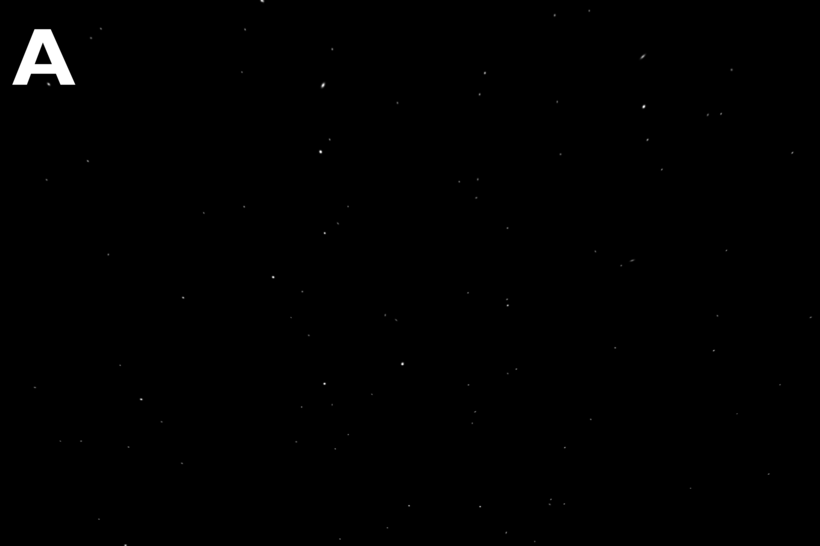Two frames (A and B) of our input sequence.

Now, let's draw the best matching star triangles between the two frames: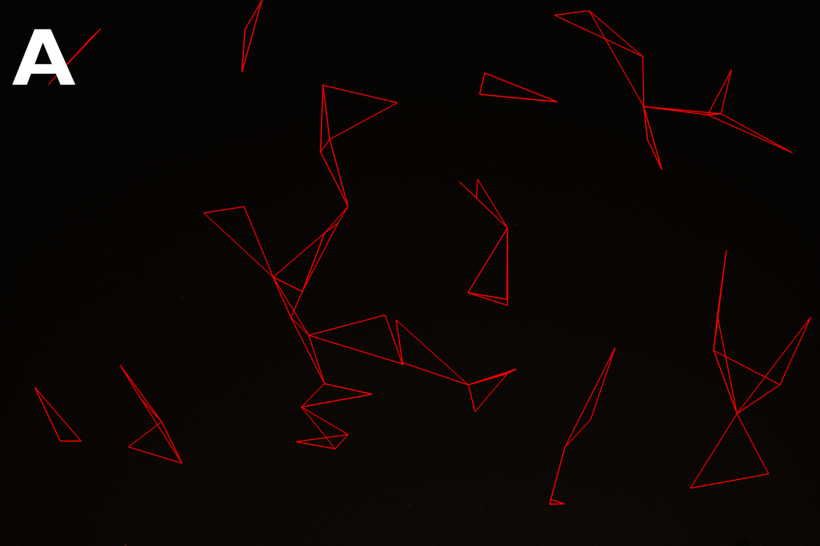Matched and tracked star constellations between the two input images.

This looks quite good! Although there are a handful of incorrectly matched triangles, the vast majority are correctly tracked across frames. Remember that all we need for a rough alignment are two pairs of correct star correspondences, and for these frames this technique delivers more than 100.

Finally, below I'm showing the rough alignment with the lowest error score, which was ultimately picked by the algorithm: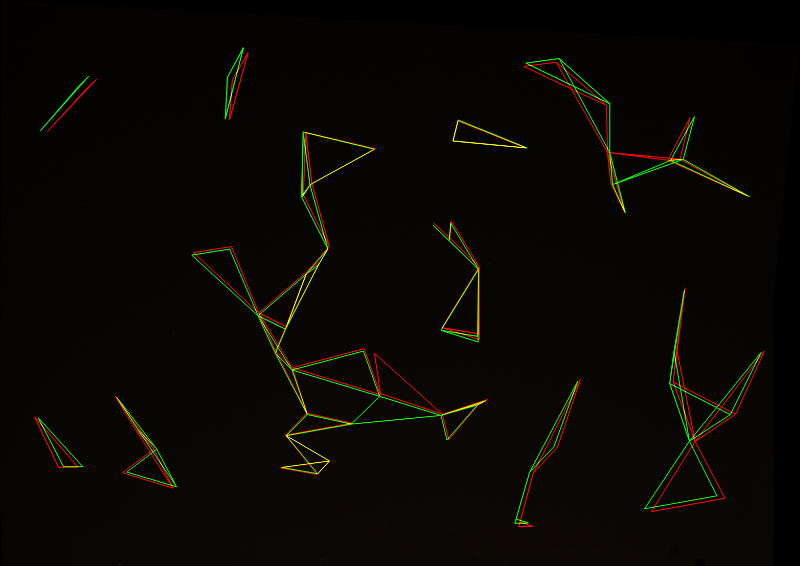Overlayed star constellations from both frames after rough alignment.

This looks quite good! Near the edges the alignment is a bit off - most likely because we don't solve for intrinsic parameters - but the fitted pose is very usable. Remember that we only need this alignment to be good enough for the fine alignment step, and this will definitely be sufficient.

### Fine star alignment

With a rough alignment in place and correspondences computed, it's time for the final alignment step. In the rough alignment step we've estimated the pose using only two pairs of correspondences. In theory, two pairs of correspondences are enough to estimate both intrinsic and extrinsic parameters; however, we have hundreds of correspondences! Somehow we want to extract intrinsic and extrinsic parameters that accomodate all correspondences and not just two - this is what fine alignment is all about.

The general term for the problem we're dealing with is an overdetermined system of equations. The go-to solution technique is least squares, which is what we will be using. I won't go into detail about least squares, but what it allows us to do is to find a set of parameters that minimizes the combined error over all aligned correspondences. If our system is linear, we can even compute the optimal solution in closed form. Unfortunately, this is not the case for our problem - rotation matrices, matrix inversion and perspective division cause significant non-linearity, and we will turn to an approximate minimization technique. The simplest such technique is gradient descent, which is what I've opted to use.

The basic idea is this: We have some formula to compute the total alignment error. Changing the intrinsic and extrinsic parameters will change the error in some way - it will either become larger or smaller. The measure of this change is the derivative of the error with respect to the parameters. If we want to reduce the error as much as possible, we simply need to follow its negative derivative.

Doing this minimization actually turns out to be quite difficult. The error we want to minimize is highly non-linear and derivatives are inconvenient and unstable to compute. Additionally, depending on which space we formulate the problem in, the minimization can be extremely unstable and produce unusable results. For production code I would recommend using a library like OpenCV, which already ships with well-engineered and tested code to do this. However, in a personal project, the pain of the learning process is quite valuable, and I've instead dug into literature to figure out how to solve this using a hand-rolled solution.

Over the course of a few weeks I implemented several optimization models, and all except one turned out to be very unreliable. Reproducing the working algorithm here would exceed the scope of this (already long) post, but there were two publications I found extremely helpful: Construction of Panoramic Image Mosaics (relevant bits: pages 10-14) and Image Alignment and Stitching. I would recommend these to anyone implementing image alignment.

With the boring bits out of the way, let's look at some actual results! Below are the same images we've seen before, animated over several iterations of the optimization algorithm. At each iteration, the pose and focal length are re-estimated and refined, leading to a better and better alignment: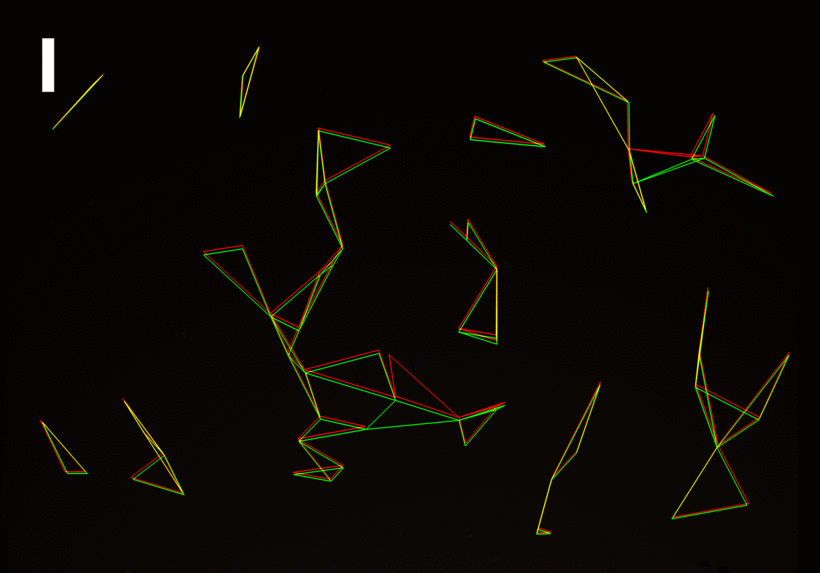Overlayed star constellations from both frames, over several iterations of the fine alignment algorithm.

Given a good enough initial guess (the rough alignment), a robust implementation of this optimization converges surprisingly quickly. Usually we're done after 10 iterations or less, rendering this the cheapest step of the program - even though it took the longest to implement.

### Merging

Now we're almost done! Using rough & fine alignment, we can align all successive images in our input sequence to each other. Doing this is called pairwise alignment, because we only align two images at a time.

It turns out we can do slightly better than this. When we do pairwise alignment, we align a star with its correspondence in one other frame. However, usually a star appears in more than just one frame - it could even appear in every frame in the sequence. To make use of this additional information, we perform one final global alignment step after we complete pairwise alignment. The global alignment optimizes all poses simultaneously to lign up all correspondences of all stars across all frames. A dizzying amount of data!

The math for global alignment is pretty much the same as for pairwise alignment, except we add some more derivatives. There is definitely not enough space here to describe the process in detail, but please see the papers linked earlier if you're interested.

With all alignments computed, we can at last produce the final output image. Doing this is quite simple: We first pick a reference camera, which in my case is always the first camera in the sequence. Then we reproject all images in the sequence into the reference camera. In other words, we iterate over all points on the image plane of the reference camera, and project those points into the image plane of every other frame in the sequence. Then we simply look up the pixel values there and average them. The output of this process is the average of the aligned images.

But enough text - let's look at the final results. Below are the first six images in the sequence, after light pollution removal and significantly boosted in brightness. I'm showing the images after alignment, and I'm averaging more and more images together: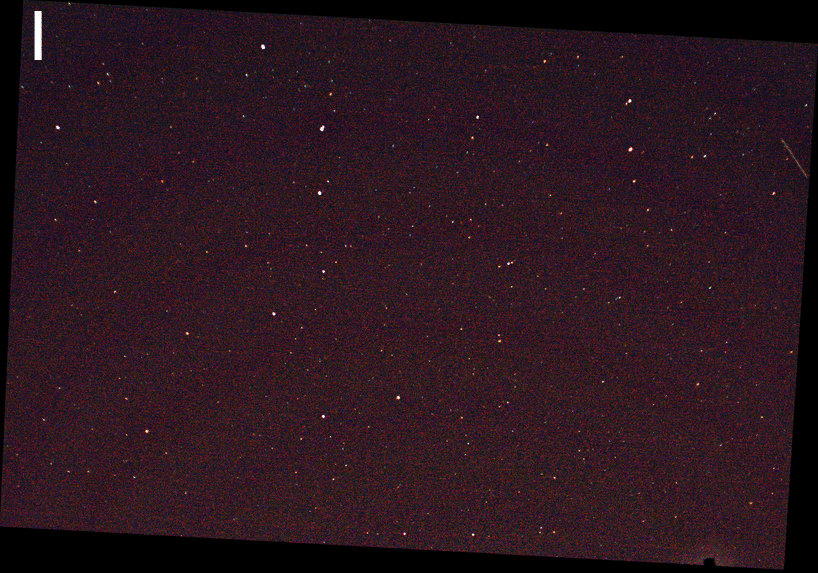Merging increasing numbers of aligned frames greatly reduces noise.

It may be hard to make out in this low-resolution version, but the images start out extremely noisy. This is because we boosted the image brightness quite a lot. However, the more images we add, the lower the noise level becomes. The cool thing is that unlike naive averaging, the stars stay perfectly sharp! Our alignment pipeline makes sure stars stay put and don't get smeared out into star trails.

Finally, here is a fast forward of the merging process of all 64 images in this sequence:

An animation of iteratively merging all images in our input sequence.

We can see that there is some additional red background tint due to parts of the light pollution we didn't catch in the first processing step. To get rid of it, we do a second light pollution subtraction after merging and tonemap the result. And we're done! Here are the full resolution results. On the top is the first image in the input sequence, on the bottom is the final merged output image: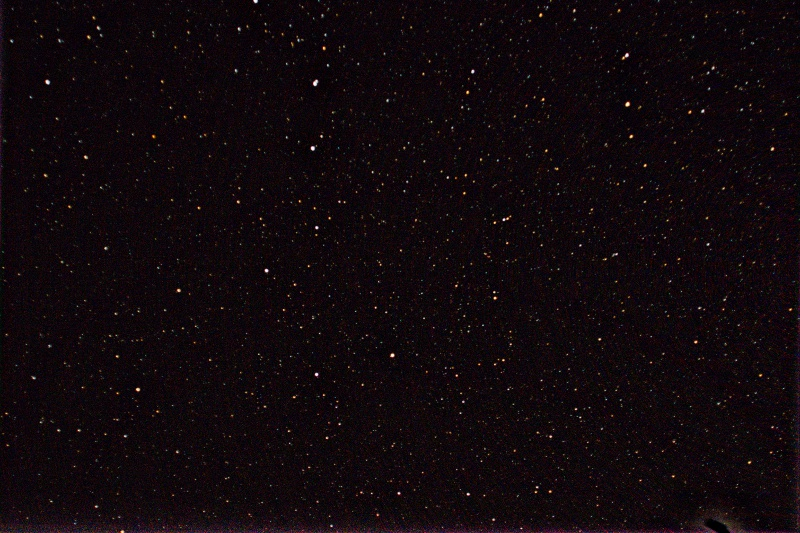The first image in the input sequence (top) and the final output after subtracting light pollution, aligning and merging (bottom).

### Conclusion

This project was a fun experiment in how much you can extract out of a low quality input image. Originally the purpose of this was just to learn more about computer vision, but I was surprised at the results obtained in the end. Although this is not production ready code, it produces nice images.

If I had more time to spend on this project, there are definitely a few things I would fix. I'm not doing any kind of dark frame subtraction, meaning that static sensor noise and dead pixels will stick around in the merged image. Additionally, I'm working with 8 bit JPEGs as input, rather than higher-precision RAW files, which limits the quality of the output a bit. Finally, all alignment is done on estimated star positions, which may be inaccurate - a final alignment step using the pixel data directly could make the results even sharper.

While working on this, I was also playing with an interesting idea for a future project: Only in a few places in the processing pipeline did we assume that all images were taken by the same camera. Additionally, we don't require input frames to be very similar to each other - the alignment algorithm is robust enough to handle arbitrary camera orientation changes between images. This opens up an interesting avenue for a crowd-sourced stargazing service. It would ask people around the globe to upload night sky images to a website, and an algorithm behind the scenes would combine all of these images into a single, high-resolution star map spanning the globe. There's definitely more work to be done on top of this project to make something like that possible, but it's an interesting idea.

### Acknowledgements

Big thanks go to the dashingly handsome Andrew Chin for providing the images in this post and sparking the initial idea for this project.